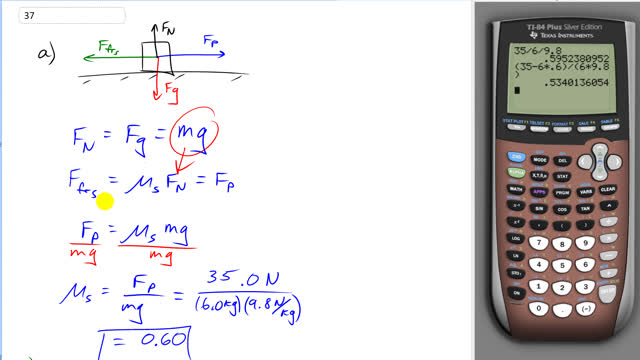## You are here

Question:

A force of 35.0 N is required to start a 6.0-kg box moving across a horizontal concrete floor.

1. What is the coefficient of static friction between the box and the floor?
2. If the 35.0-N force continues, the box accelerates at $0.60 \textrm{ m/s}^2$. What is the coefficient of kinetic friction?

Giancoli, Douglas C., Physics: Principles with Applications, 7th Ed., ©2014. Reprinted by permission of Pearson Education Inc., New York.
The question will be visible after logging in, as required by Pearson Education Inc.

1. $0.60$
2. $0.53$